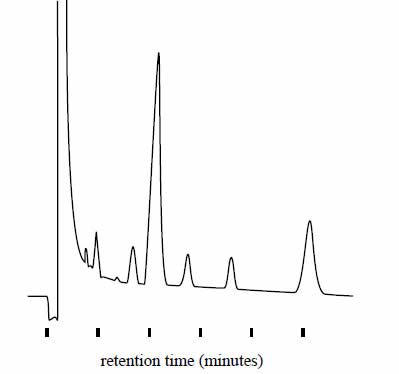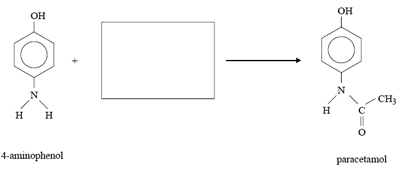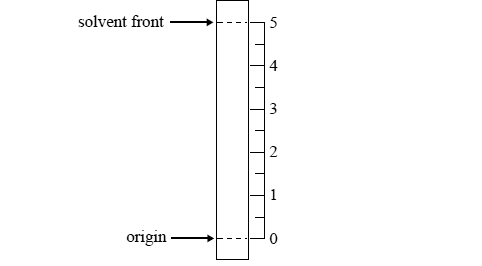Chromatography exercise (2008) Deadly diseases such as tetanus, botulism and gangrene are caused by related groups of bacteria (clostridium genus) found in the soil. Each group of bacteria produces specific volatile fatty acids. These fatty acids can be identified using gas chromatography by comparison with a control chromatogram of known standards. The following gas chromatogram is of the fatty acids produced by one such group of bacteria.The identity of the fatty acids can be determined by measuring A. their retention times. B. the temperature of the column. C. the fl ow rate of the carrier gas. D. the area under each of the peaks. Solution The relative amount of each of the fatty acids can be determined by measuring A. their retention times. B. the temperature of the column. C. the flow rate of the carrier gas. D. the area under each of the peaks. SolutionParacetamol is a commonly used painkiller. The equation below shows one method of preparing paracetamol.a. In the box provided in the equation above, give the formula of a possible reagent that can be used in this synthesis. Solution b. A student uses thin layer chromatography (TLC) to analyse the products of this preparation of paracetamol. For the stationary and mobile phases used for this analysis, the Rf of paracetamol is 0.4. On the diagram of a TLC plate, on the right, use a horizontal line to mark the spot where paracetamol would appear in such an analysis. Solution4-aminophenol adsorbs less strongly than paracetamol onto the stationary phase of this TLC plate. Predict whether the Rf value of 4-aminophenol in this analysis is greater or smaller than that of paracetamol, giving a reason for your choice. Solution